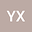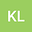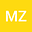New upper bounds of cumulative coherence for $\ell_{1-2}$-minimization in compressed sensing
•••• +1
• Youwei Xie,
• Kaihao Liang,
• Meijiao Zhang,
• Shaohua Xie
Youwei Xie
Quanzhou University of Information Engineering
Author ProfileKaihao Liang
Zhongkai University of Agriculture and Engineering
Author ProfileMeijiao Zhang
Quanzhou University of Information Engineering
Author ProfileShaohua Xie
Sun Yat-Sen University

Corresponding Author:[email protected]

Author Profile## Abstract

This paper focuses on the compressed sensing $\ell_{1-2}$-minimization problem and develops new bounds on cumulative coherence $\mu_1(s)$. We point out that if cumulative coherence $\mu_1(s-1)$ and $\mu_1(2s-1)$ satisfy $(\ref{eq:EqNo2})$, or cumulative coherence $\mu_1(2s-1)$ satisfies $(\ref{eq:EqNo11})$ then the sparse signal can via $\ell_{1-2}$-minimization problem stably recover in noise model and exact recovery in free noise model.